Back to the main index

# Natural image statistics¶

Part of the introductory series to using Python for Vision Research brought to you by the GestaltReVision group (KU Leuven, Belgium).

In this part we will capitalize on the basics you learned in Part 1 to build a real working experiment.

Authors: Bart Machilsen, Maarten Demeyer
Year: 2014
Copyright: Public Domain as in CC0

In :
# Import all the necessary packages
import os, urllib2, json, io

import numpy as np
from PIL import Image
import matplotlib.pyplot as plt
from matplotlib.colors import hsv_to_rgb

# Prepare directories
imagedir = os.path.join(os.getcwd(),'images')

if not os.path.isdir('results'):
os.makedirs('results')
if not os.path.isdir(os.path.join(imagedir,'panoramio')):
os.makedirs(os.path.join(imagedir,'panoramio'))


# Fourier transform¶

Fourier theorem (simplified): Any signal can be expressed as a sum of sine waves. A Fourier transformation therefore decomposes a signal into a series of sine waves, each with their own amplitude, frequency and phase.

The Fast Fourier Transform is a special case of this, decomposing equally spaced samples from the signal into an equal number of equally spaced discrete frequency components. This is an approximation of a real Fourier Transform.

## One Dimension¶

In :
# Frequency and sampling rate
ph = np.pi/2
freq = 20
amp = 1
n_samples = 200

# Define 1-D signal
x = np.linspace(0,1,n_samples)
y = np.sin(ph + (2*np.pi*freq*x))*amp

# Plot 1-D signal
plt.figure(figsize=(10,1))
plt.plot(x,y,'b-')
plt.show()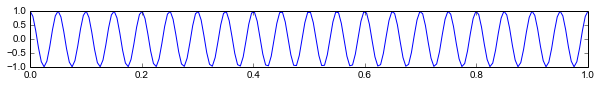You could imagine this wave as a sound wave. This one-frequency wave would be a pure tone. If this would be a 'Do', then doubling the frequency would give a 'Do' but one octave higher.

In :
def one_d_fft(y):

# Perform the actual FFT
F = np.fft.fft(y)

# The full spectrum F consists of complex numbers ordered by frequency
# So, extract frequency, amplitude, phase information like this
fft_freq = np.fft.fftfreq(len(F))*n_samples
fft_amp = np.abs(F)/n_samples
fft_ph = np.angle(F)

# Plot the result
plt.figure(figsize=(15,5))
plt.subplot(1,2,1)
plt.plot(fft_amp,'r.')
xt = np.linspace(0,len(fft_freq), 5).astype(int)
plt.xticks(xt, 1+fft_freq[xt-1].astype(int))
plt.xlabel("Frequency",fontsize=20), plt.ylabel("Amplitude",fontsize=20)

plt.subplot(1,2,2)
plt.plot(fft_ph,'b.')
plt.xticks(xt, 1+fft_freq[xt-1].astype(int))
plt.xlabel("Frequency",fontsize=20), plt.ylabel("Phase",fontsize=20)
plt.show()

return fft_amp, fft_ph

fft_amp, fft_ph = one_d_fft(y)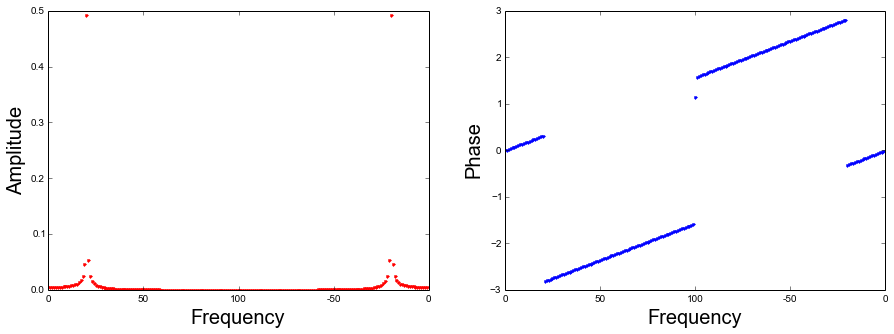Note the scale of the axes.

• X-axis can't be higher than half the number of samples because the Nyquist frequency, i.e. half the sampling rate, is the maximal one that can be extracted. In the case of real numbers, the spectrum is symmetrical around the Nyquist frequency.
• Y-axis of Amplitude divides the total amplitude over both symmetrical components.
• Y-axis of Phase is poorly informed here, but you'll notice that the average of the sudden jump equals the actual phase value.

The inverse Fourier transform reconstructs the original signal.

In :
# Now do the inverse Fourier transofrm
i_F = fft_amp*n_samples * np.exp(1j*fft_ph)
i_y = np.fft.ifft(i_F).real

# Plot reconstructed 1-D signal
plt.figure(figsize=(10,1))
plt.hold(True)
plt.ylim([-1,1])
plt.plot(x,y,'b-')
plt.plot(x,i_y,'r-')
plt.hold(False)
plt.show()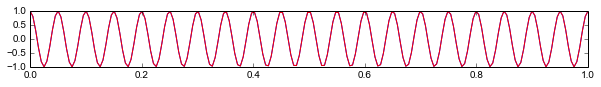More complex signals will show a more complex pattern of amplitudes and phases. For instance, this is the Fourier spectrum of a sum of three sine waves.

In :
ph1 = np.pi/2; ph2 = 0; ph3 = 5*np.pi/4
freq1 = 9; freq2 = 20; freq3 = 35
amp1 = 1; amp2 = 1.5; amp3= 0.75
n_samples = 200

x = np.linspace(0,1,n_samples)
y1 = np.sin(ph1 + (2*np.pi*freq1*x))*amp1
y2 = np.sin(ph2 + (2*np.pi*freq2*x))*amp2
y3 = np.sin(ph3 + (2*np.pi*freq3*x))*amp3
ys = y1+y2+y3

plt.figure(figsize=(10,1))
plt.hold(True)
plt.plot(x,y1,'b-'); plt.plot(x,y2,'r-'); plt.plot(x,y3,'g-')
plt.plot(x,ys,'k-', linewidth=2)
plt.hold(False)
plt.show()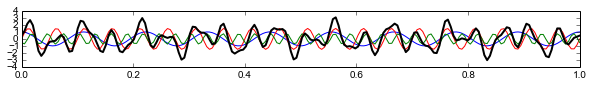In :
fft_amp, fft_ph = one_d_fft(ys)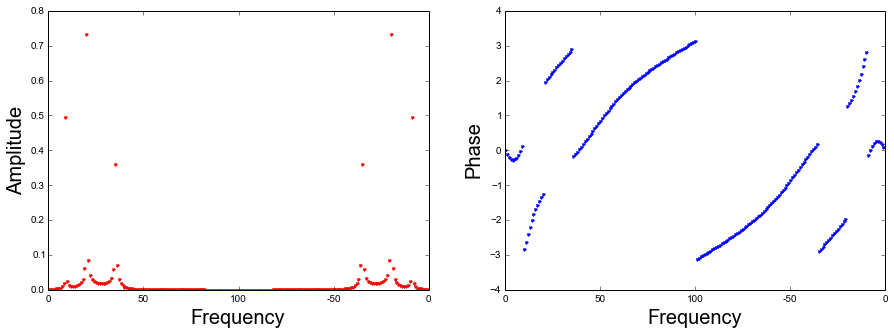Note that this is how MP3 compression works: components that are less relevant (e.g. low amplitude, or low sensitivity to its frequency) are removed from the Fourier transform to reduce the total amount of data that needsto be saved.

## Two Dimensions¶

### fft2¶

Fourier transforms in two dimensions work similarly, but naturally return 2D spectra as well. In case of images, these spectra will each have the size of a full image.

In :
def do_fourier_transform(img):

# Do the fft
F = np.fft.fft2(img)

# Center the spectrum on the lowest frequency
F_centered = np.fft.fftshift(F)

# Extract amplitude and phase
A = np.abs(F_centered).real
P = np.angle(F_centered).real

# Return amplitude, phase, and the full spectrum
return A, P, F

In :
plt.figure(figsize=(15,5))

plt.subplot(1,4,1), plt.imshow(img), plt.axis('off')
img = np.mean(img,axis=2)
plt.subplot(1,4,2), plt.imshow(img, cmap='gray'), plt.axis('off')

A, P, F = do_fourier_transform(img)

plt.subplot(1,4,3), plt.imshow(np.log(A), cmap='gray'), plt.axis('off')
plt.subplot(1,4,4), plt.imshow(P, cmap='gray'), plt.axis('off')

plt.show()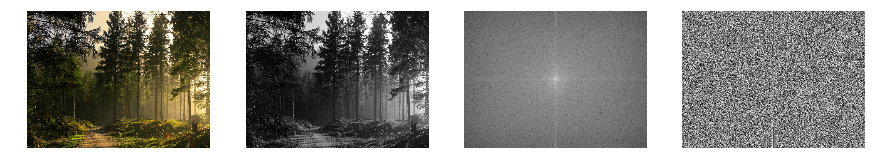You may notice a few things: There is a cardinal bias, and the lower frequencies have higher amplitudes. This is a fairly general pattern that is shared by many natural images. The real image information is in the more complex phase spectrum.

### Rotational average and 1/f spectrum¶

To illustrate the relation between frequency and amplitude more clearly, we will take a rotational average, regardless of orientation.

In :
def get_band_mask(spectrum, band, n_bands):
"""Select a circular frequency band from the spectrum"""
# Get image coordinates, and center on 0
x,y = np.meshgrid(range(spectrum.shape),range(spectrum.shape))
x = x - np.max(x)/2
y = y - np.max(y)/2

# Compute distances from center

# Compute the min and max frequencies of this band
bw = np.amin(spectrum.shape)/(n_bands*2)
freqs = [0+bw*band,bw+bw*band]

msk = np.zeros(spectrum.shape, dtype=bool)

# Do not include the zero-th frequency (overall luminance)
msk[x.shape/2,y.shape/2] = False
return msk

# An illustration of what this does
plt.figure(figsize=(15,5))
plt.subplot(1,7,1)
plt.imshow(np.log(A),cmap='gray')
plt.axis('off')

n_bands = 6
for band in np.arange(n_bands):
plt.subplot(1,n_bands+1,band+2)
plt.imshow(msk*np.log(A),cmap='gray')
plt.axis('off')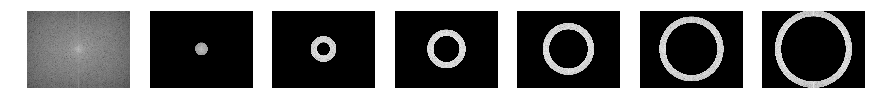In :
def get_rotational_average(A, n_bands):
"""Average over the contents of n frequency bands"""
res = []
for band in np.arange(n_bands):
res.append(np.mean(A[msk].flatten())/A.size)
return np.array(res, dtype=float)

In :
rotavg = get_rotational_average(A, 20)

plt.figure(figsize=(5,5))
plt.plot(range(1,len(rotavg)),rotavg[1:],'k-')
plt.xlabel('Frequency Band')
plt.ylabel('Mean Amplitude')
plt.show()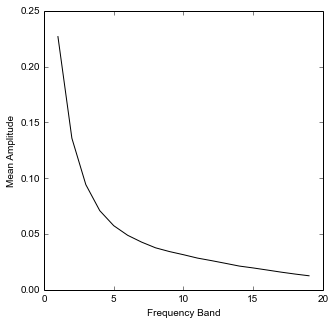Thus: Fourier amplitudes decrease exponentially as the inverse of the corresponding frequencies. It is said that natural images have a 1/f spectrum.

### Frequency filtering of images¶

Fourier analysis is easily extended to the low-pass, high-pass or bandpass filtering of images. For instance:

In :
def do_inverse_fourier_transform(A, P):
"""Reconstruct the image from amplitude and phase spectrum"""
F_centered = A * np.exp(1j*P)
F = np.fft.ifftshift(F_centered)
img = np.fft.ifft2(F).real
return img

In :
# Do the Fourier transform, copy the amplitude spectrum
A, P, F = do_fourier_transform(img)
Af = A.copy()

# Compute one frequency at each pixel
fx = np.fft.fftshift(np.fft.fftfreq(A.shape))
fy = np.fft.fftshift(np.fft.fftfreq(A.shape))
fx,fy = np.meshgrid(fy,fx)
freq = np.hypot(fx,fy)

# Filter and reconstruct
bandpass = (freq>0) * (freq<0.05)
Af[~bandpass] = 0
f_img = do_inverse_fourier_transform(Af, P)

# Show result
plt.figure(figsize=(10,10))
plt.imshow(f_img,cmap='gray')
plt.axis('off')
plt.show()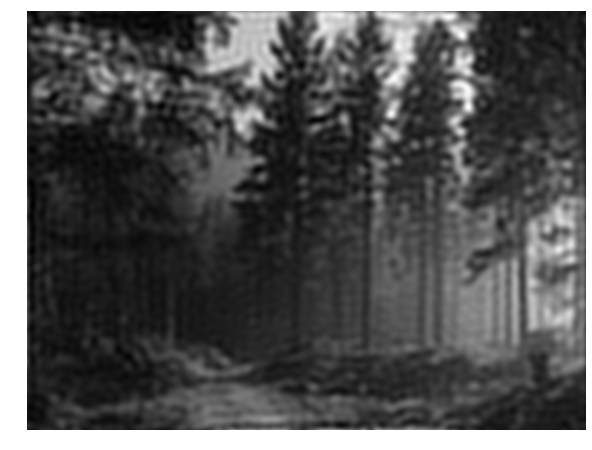You might notice the ringing artefacts familiar from JPEG compressed images, exaggerated here because of the simplified method. This is indeed how the compression algorithm works: higher frequency components are removed.

### Whitening and white noise¶

Amplitude and frequency can be decorrelated by a process called whitening. Quite simply, we apply a uniform amplitude spectrum and retain the phase spectrum.

In :
# Generate and apply a uniform amplitude spectrum
wA = np.ones(A.shape)
w_img = do_inverse_fourier_transform(wA, P)

plt.figure(figsize=(10,10))
plt.imshow(w_img, cmap='gray'), plt.axis('off')
plt.show()